Transport Properties in High Electric Fields

 Remarks Referens Electron saturated drift velocity 4H-SiC Vsat = 8.0 x 106 cm/s 300 K,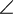(E, c) = 8° towards <11-20> Vassilevski et al. (2000) 4H-SiC Vsat = 7.5 x 106 cm/s 460K,(E,c) = 8° towards <11-20> Vassilevski et al. (2000) 4H-SiC Vsat = 3.3 x 106 cm/s RT, E||c, saturation field = 1.55x105 V/cm, n+-p-n+ SiC structures Sankin et al. (2000) 8H-SiC Vsat = 1.0 x 106 cm/s RT, E||c, saturation field = 1.35x105 V/cm n+-p-n+ SiC structures Sankin et al. (2000) 15R-SiC Vsat = 1.2 x 106 cm/s RT, E||c, saturation field = 1.35x105 V/cm n+-p-n+ SiC structures Sankin et al. (2000) 21R-SiC Vsat = 4.4 x 103 cm/s RT, E||c, saturation field = 1.35x105 V/cm n+-p-n+ SiC structures Sankin et al. (2000)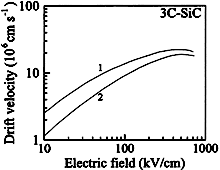3C-SiC. Calculated electron steady-state drift velocity vs. electric field at 2 different temperatures T. Total concentration of ionized dopants is 1.5x1018cm-3 1 - T = 300 K; 2 - T = 600 K. Mickevicius & Zhao (1998)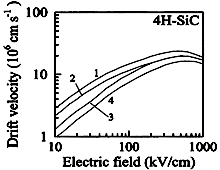4H-SiC. Calculated electron steady-state drift velocity vs. electric field Doping concentration Nd = 1.5 x 1018 cm-3. 1 - T = 300 K; electric field E||c; 2 - T = 300 K, electric field E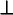c; 3 - T = 600 K, electric field E||c; 4 - T = 600 K, electric field Ec. Mickevicius & Zhao (1998)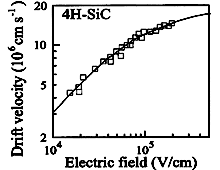4H-SiC. Experimental electron steady state drift velocity vs. electric field T = 300 K. Electron concentration 1.4 x 1017 cm-3. Mobility in the low electric field is 400 cm2/V·s. Khan & Cooper (1998)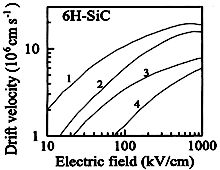6H-SiC. Calculated electron steady-state drift velocity vs. electric field Doping concentration Nd = 1.5 x 1018 cm-3. 1 - T = 300 K; electric field EcE||c; 2 - T = 300 K, electric field E||c; 3 - T = 600 K, electric field Ec; 4 - T = 600 K, electric field E||c. Mickevicius & Zhao (1998)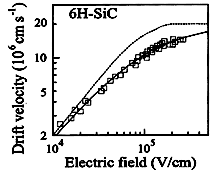6H-SiC. Experimental electron steady state drift velocity vs. electric field T = 300 K. Electron concentration 1.2 x 1017 cm-3. Mobility in the low electric field is 200 cm2/V·s. Khan & Cooper (1998)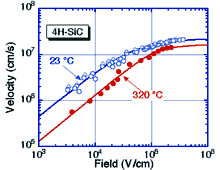6H-SiC. Experimental electron steady state drift velocity vs. electric field at two temperatures. Solid lines are generated using the equation V(E) = µ E /[1+ ( µ E / Vsat )a ]1/a with parameters: T = 23 K.   µ = 450 cm2/Vs,   Vsat= 2.2x107 cm/s,   a =1.2; T = 320 K. µ = 130 cm2/Vs,   Vsat= 1.6x107 cm/s,   a =2.2. Khan & Cooper (2000) Contact authors: James A. Cooper. Also see High Field Transport.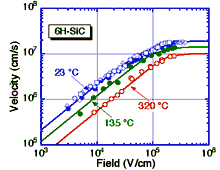6H-SiC. Experimental electron steady state drift velocity vs. electric field at two temperatures. Solid lines are generated using the equation V(E) = µ E /[1+ ( µ E / Vsat )a ]1/a with parameters: T = 23 K.   Mobility µ = 215 cm2/Vs,   Vsat= 1.9x107 cm/s,   a = 1.7; T = 135 K. Mobility µ = 120 cm2/Vs,   Vsat= 1.4x107 cm/s,   a = 2.5; T = 320 K. Mobility µ = 56 cm2/Vs,     Vsat= 1.0x107 cm/s,   a = 4.0. Khan & Cooper (2000) Contact authors: James A. Cooper. Also see High Field Transport.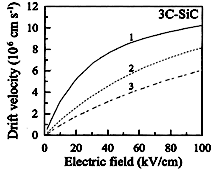3C-SiC. Calculated Hole steady-state drift velocity vs. electric field applied along (100) direction (relatively low fields) T = 300 K; Impurity concentration: 1 - 0.1 x 1018cm-3; 2 - 1.0 x 1018cm-3; 3 - 7.0 x 1018cm-3. Bellotti et al. (1999)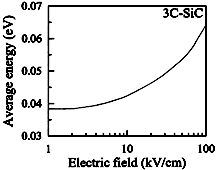3C-SiC. Calculated average Hole energy vs. electric field applied along (100) direction (relatively low fields). T = 300 K. Bellotti et al. (1999)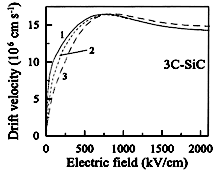3C-SiC. Calculated Hole steady-state drift velocity vs. electric field applied along (100) direction (high fields). T = 300 K; Impurity concentration: 1 - 0.1 x 1018cm-3; 2 - 1.0 x 1018cm-3; 3 - 7.0 x 1018cm-3. Bellotti et al. (1999)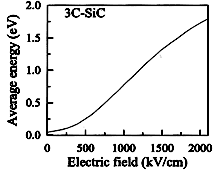3C-SiC. Calculated average Hole energy vs. electric field applied along (100) direction (high fields). T = 300 K. Bellotti et al. (1999)